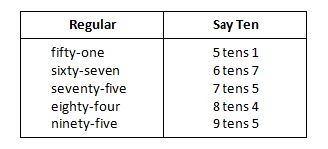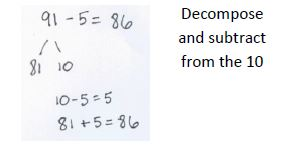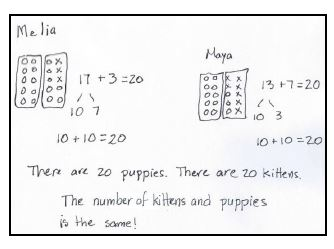2nd Module 1--Sums and Differences to 20

Module 1 Topics
Topic A reactivates students' Kindergarten and Grade 1 learning. Student remember their "make ten" facts. They use ten-frame cards and number bonds of ten to move from concrete to pictorial to abstract representations.They will use mental strategies to add and subtract within 20.mental strategiesnumber bondssay ten counting

Topic B- Students use decomposing strategies to add and subtract within 20. They will use the ten- structure to reason about making a ten to add and subtract. Students will use math drawings to solve problems and understand the relationship between problems.

Topic C calls on students to review strategies to add and subtract within 100.Decompose and add to make the tenRDWW  is a four step problem-solving process. The steps are as follows: